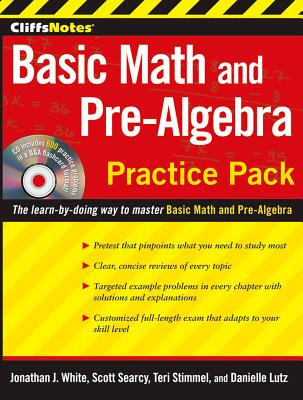### CliffsNotes Basic Math and Pre-Algebra Practice Pack with CD (Paperback)

Cliffs Notes, 9780470533499, 264pp.

Publication Date: February 26, 2010

List Price: 18.99*
* Individual store prices may vary.

#### Description

Basic Math and Pre-Algebra

Pretest

Helps you pinpoint where you need the most help and directs you to the corresponding sections of the book

Topic Area Reviews

The basics

• Whole numbers
• Fractions
• Decimals
• Percents
• Integers and rational numbers
• Powers, exponents, and roots
• Powers of ten and scientific notation
• Measurements
• Charts and graphs
• Probability and statistics
• Variables, algebraic expressions, and simple equations

Appendix

• Arithmetic and geometric sequences
• Predicting the next term of a sequence

Glossary

• Defines terminology used in the book

Customized Full-Length Exam

• Covers all subject areas